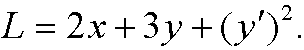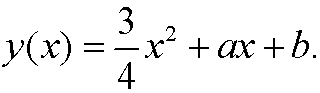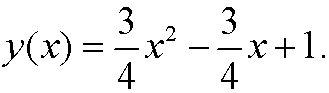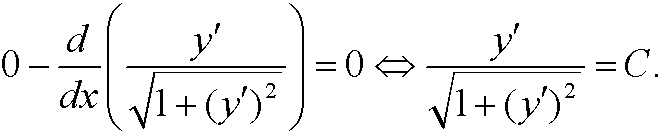# 6. Two solved problems.

Example 10: Find the extremal to the functionalSolution: Here we haveThis implies thatand Euler’s equation therefore becomesTwo integrations giveWith the boun dary conditions inserted the solution becomes<>Example 11: (Compare example 4.) Find the extremal to the functional

<>Solution: The lagrangian isand Euler’s equation thus becomesIf we solve fory  we getwhereWith the boundary conditions inserted we then get